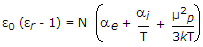# Electronics and Communication Engineering - Materials and Components

### Exercise :: Materials and Components - Section 3

11.

The resistivity of intrinsic semiconductors, at room temperature, is of the order of

 A. 1 Ω-m B. 1000 Ω-m C. 106 Ω-m D. 10-3 Ω-m

Explanation:

No answer description available for this question. Let us discuss.

12.

Assertion (A): Ferromagnetic materials possess ferromagnetic properties only when temperature is less than paramagnetic curie temperature.

Reason (R): For a ferromagnetic material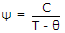for T > θf.

 A. Both A and R are true and R is correct explanation of A B. Both A and R are true but R is not correct explanation of A C. A is true but R is false D. A is false but R is true

Explanation:

No answer description available for this question. Let us discuss.

13.

In terms of classical representation of electrons

 A. an atom is essentially empty B. an atom is essentially full C. an atom may be essentially empty or full depending on the material D. an atom may be essentially empty or full depending on temperature

Explanation:

No answer description available for this question. Let us discuss.

14.

To produce magnetic flux, mmf is necessary.

 A. True B. False

Explanation:

No answer description available for this question. Let us discuss.

15.

For a polyatomic gas, the equation relating dielectric constant ∈r with molecular properties is

 A.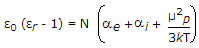B.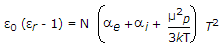C.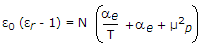D.